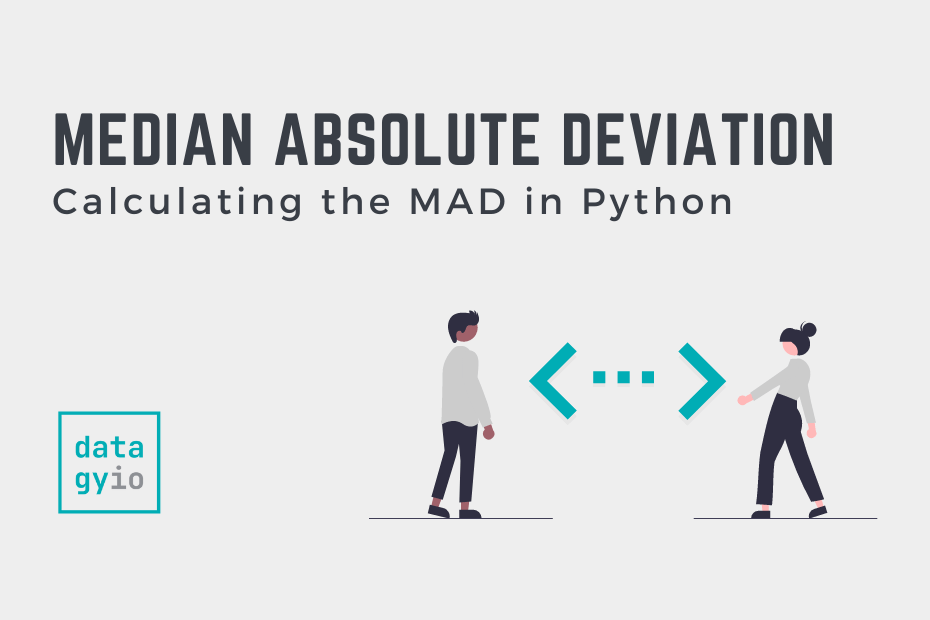# SciPy## How to Calculate the Median Absolute Deviation in Python

The median absolute deviation (MAD), is a robust statistic of variability that measures the spread of a dataset. In this tutorial, you’ll learn how to use Python to calculate the median absolute deviation. By the end of this tutorial, you’ll… Read More »How to Calculate the Median Absolute Deviation in Python## Calculate Manhattan Distance in Python (City Block Distance)

In this tutorial, you’ll learn how to use Python to calculate the Manhattan distance. The Manhattan distance is often referred to as the city block distance or the taxi cab distance. The Manhattan distance can be a helpful measure when… Read More »Calculate Manhattan Distance in Python (City Block Distance)## Calculate Hamming Distance in Python (with Examples)

In this tutorial, you’ll learn how to calculate the hamming distance in Python, using step-by-step examples. In machine learning, the Hamming distance represents the sum of corresponding elements that differ between vectors. By the end of this tutorial, you’ll have… Read More »Calculate Hamming Distance in Python (with Examples)## Calculate the Pearson Correlation Coefficient in Python

In this tutorial, you’ll learn how to calculate the Pearson Correlation Coefficient in Python. The tutorial will cover a brief recap of what the Pearson correlation coefficient is, how to calculate it with SciPy and how to calculate it for… Read More »Calculate the Pearson Correlation Coefficient in Python## How to Calculate a Z-Score in Python (4 Ways)

In this tutorial, you’ll learn how to use Python to calculate a z-score for an array of numbers. You’ll learn a brief overview of what the z-score represents in statistics and how it’s relevant to machine learning. You’ll then learn… Read More »How to Calculate a Z-Score in Python (4 Ways)